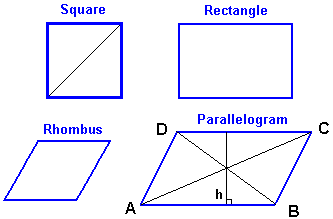## The Square (Geometry) Calculator

 edge units perimeter units diagonal units area square units
significant figures:

A Square is a flat shape, The square has 4 equal sides and 4 internal angle.

Opposite sides are parallel

Each Internal angle is 90°

### Square formula:

Area = a2 = a × a

Perimeter = 4a

Diagonal "d" = a × √2For example, When side =2, then the area = 4, perimeter = 8, diagonal = 2.83.

For example, When perimeter = 12, then the side = 3, area = 9, diagonal = 4.24.

The calculation is made easier here.

Thinkcalculator.com provides you helpful and handy calculator resources.